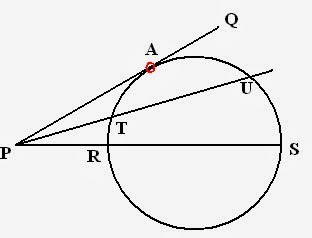I have already discussed some part of geometry in my previous sessions. Both are related to triangles and their properties. Also, I have discussed some Important Questions of geometry. Today I will discuss circles and its properties in exam point of view. Firstly I will tell you some terms related to figure of circle.

## Basic Terminologies• Centre: 'O' is a centre of the circle i.e. fixed point in the circle.
• Radius: OM is a radius of circle i.e.from centre to the boundary of circle.
• Diameter: Double of radius. In figure, XY is a diameter.
• Circumference: 2pir i.e. distance around the circle.
• Secant: A line which intersect the circle at two points. In figure, line PQ intersect circle at AB, So PQ is a secant.
• Tangent: Any line which touches the circle at only one point is tangent of circle. TU is a tangent of circle.
• Chord: Line whose end points touches the circle . RS is a chord of circle.
• Segment: Chord divides the circle into two parts. one major and other minor. Both parts are known as Segments. In figure, yellow region is a minor segment.

## Important Results of Circles

#### Area of Circle

If r is radius of circle, then area of circle = pi r^2

Example1: If area of circle is 314, then what will be the radius of circle?
Solution: Area = pi r^2
⇒ 200 = pi r^2
⇒ r^2 = 314/3.14 (pi = 3.14)
⇒ r = 10

#### Secants

In a figure given below, PA is a tangent and PRS is a secant, then
PR times PS = PA^2 (Result for one tangent and one secant)If there are two secants, for example in figure, PRS and PTU are secants, then result followed will be:
PT times PU = PR times PS

#### Angles of same segmentYellow part is a segment of circle.
Always remember that:
• Angles subtended by same segments are always equal. In figure, angle P and Q are equal, as they are subtended by same segment.
• Angle subtended by segment at the centre is twice the angle subtended at the boundary Angle O = 2 angle P = 2 angle Q.
Study the following figure:Above figure shows that, If OC is a perpendicular to AB, C will be the mid-point of AB
i.e. AC = CB and vice-versa.

#### Alternate segment theoremIf AB is tangent, then alternate angles will be equal in given figure.

I hope you understand the above important results. Will soon update more articles on Geometry. Thanks for visiting.
Also Check: Data Interpretation
How to prepare for Quantitative aptitude section
Geometry(Part-3) : Circles and their propertiesReviewed by Jasleen Behl on Sunday, March 16, 2014 Rating: 5